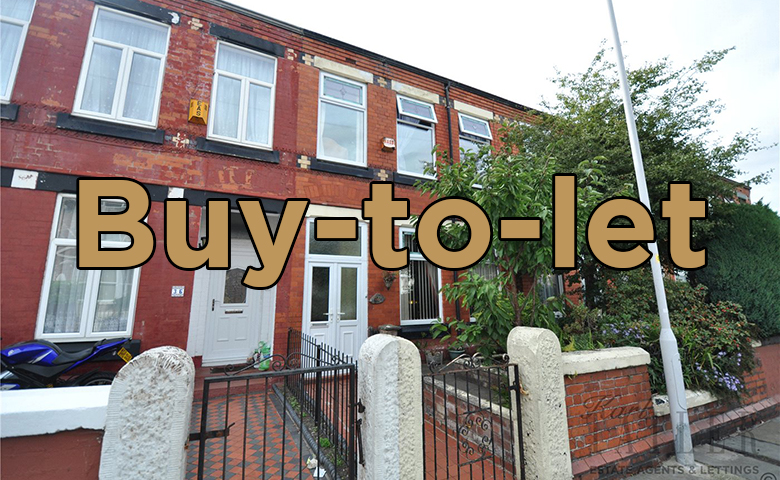Tue 10 Oct 2017 View all news articles

# Working out the real yield on your buy to let investmentWhen looking at a buy to let investment many people fall into the trap of assuming the gross rental income as a percentage of the cost of a property gives them the annual return. This figure actually gives the gross rental yield which can be very different from the annual return. Let us look at these figures in more detail.

PURCHASE OF A £100,000 PROPERTY
If you acquire a buy to let property for £100,000 then you should expect to put down a deposit of around £25,000. So you have £25,000 of equity in the property and £75,000 of finance. Let us assume that the property could be rented out for £500 a month which equates to an annual rental income of £6000. This is where the difference between gross yield and annual return comes in because simply dividing the rental income by the total cost of the property gives you a gross rental yield of 6%.

However, what you are really looking for is the annual return on your investment which is actually different from the gross rental yield.

A traditional buy to let mortgage will involve the repayment of initial capital at the end of the mortgage. A buy to let investor will simply cover interest payments on a monthly basis with the final capital repayment remaining constant throughout. In the above example there would be a final capital repayment of £75,000.

Let’s assume that a buy to let mortgage was agreed at an interest rate of 5% which equates to £312.50 per month or £3750 a year. So we have a gross rental income of £6000 and annual interest payments of £3750 leaving a surplus of £2250 a year. If we assume that there is around £2000 in expenses when acquiring the property this gives us an initial investment of £27,000 – including the deposit.

ANNUAL RETURN
The annual return is simply the difference between rental income and interest payments, in this instance £2250, as a percentage of the initial investment of £27,000. So, for this particular example the annual return is 8.33% which is greater than the gross rental yield of 6% although there may be ongoing cost to take into consideration. However, this does give you an idea of the difference between the annual return (on your investment) and the gross rental yield on the property.